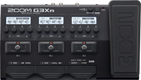# Banbeg

Discussion in 'Zoom G3n/G3Xn/G5n' started by Banbeg, Dec 31, 2022.

1. BanbegDevice: Zoom G3Xn
Firmware: 2.20

Name on device: Fishy
Optimized for: Guitar Amp

Effects chain:SAMANTHA FISH BLUES

Effect: "ZNR" (Dynamics), active - "yes"
"Detect" = EFXIN
"Depth" = 100
"Threshold" = 30
"Decay" = 0

Effect: "Squeak" (Overdrive / Distortion), active - "no"
"Gain" = 17
"FLTR" = 70
"Volume" = 100
"DryMx" = 35

Effect: "DYN Drive" (Overdrive / Distortion), active - "yes"
"Gain" = 49
"Tone" = 66
"Mode" = STACK
"Volume" = 67

Effect: "Gt GEQ 7" (Filter), active - "yes"
"100 Hz" = 0.0
"200 Hz" = 0.0
"400 Hz" = 0.0
"800 Hz" = 1.5
"1.6 kHz" = 1.5
"3.2 kHz" = 0.0
"6.4 kHz" = 0.0
"Volume" = 80
"100 Hz" = 0.0
"200 Hz" = 0.0
"400 Hz" = 0.0
"800 Hz" = 0.0
"1.6 kHz" = 0.0
"3.2 kHz" = 0.0
"6.4 kHz" = 0.0
"Volume" = 100
"Parameter set" = A

Effect: "Spring" (Reverb), active - "yes"
"PreD" = 1
"Decay" = 6
"Mix" = 47
"Tail" = On

Patch Volume: 100